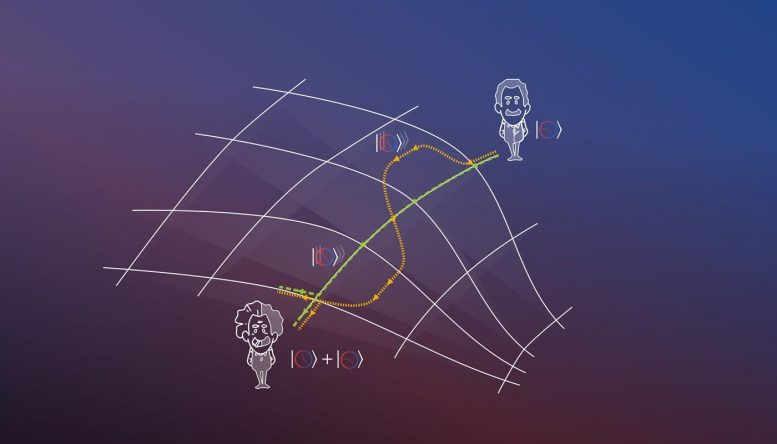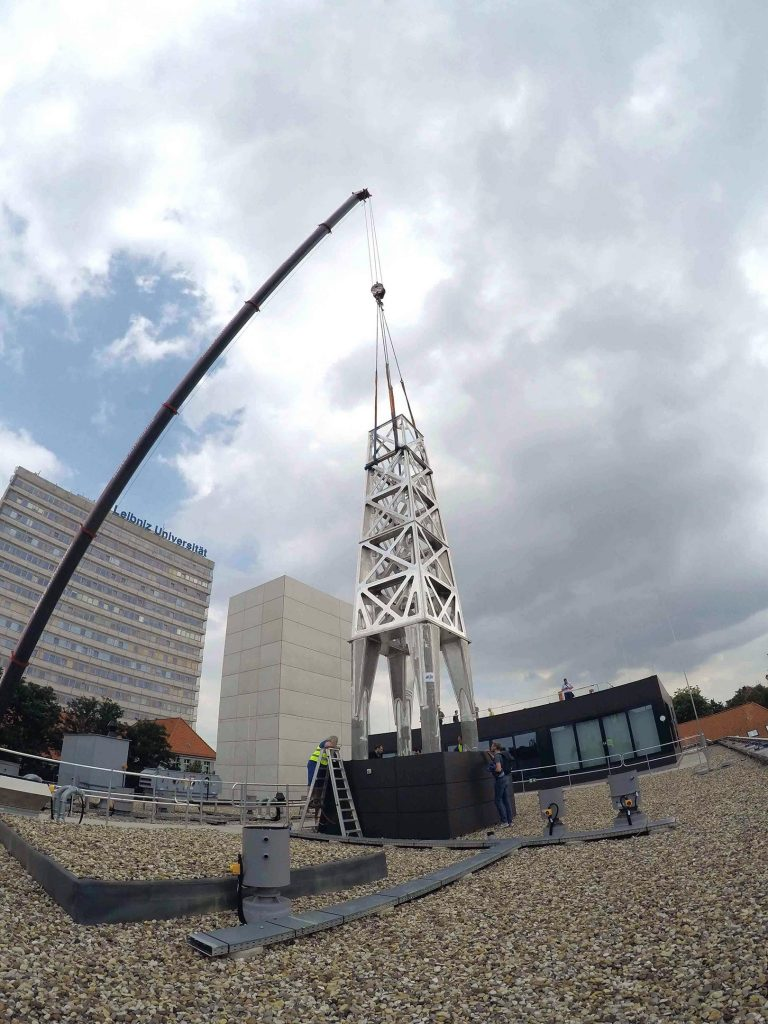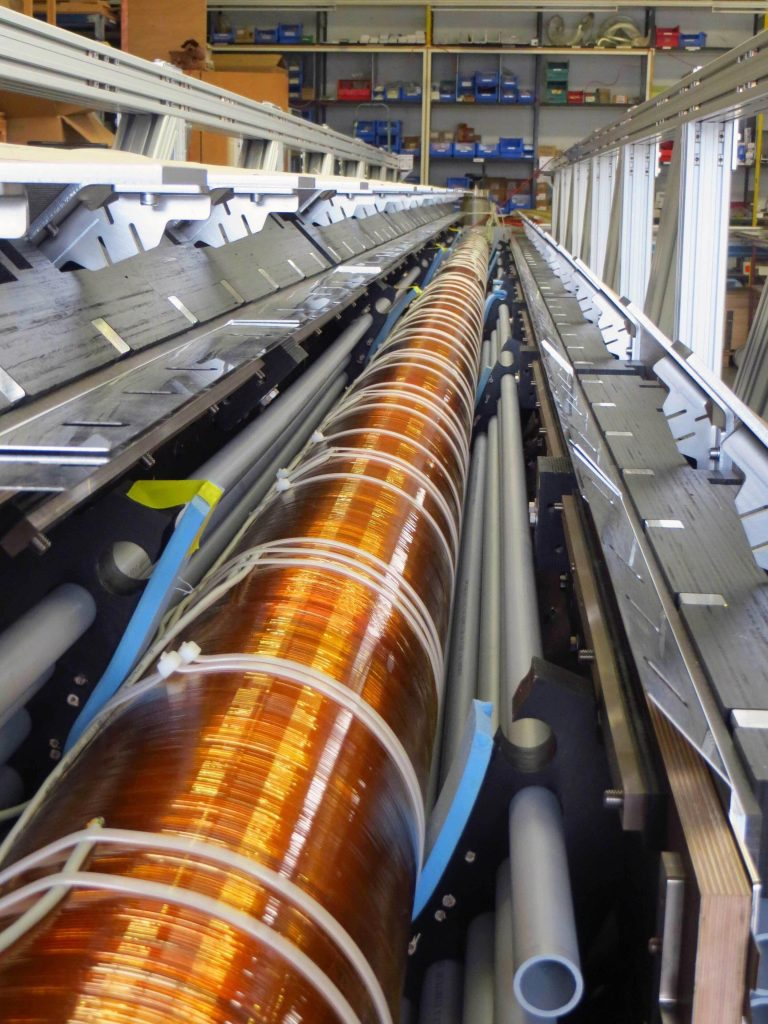# Physicists Put Einstein to the Test With a Quantum-Mechanical Twin ParadoxGraphic illustration of the quantum-mechanical variant of the twin paradox. Credit: Ulm University

### Physicists from Ulm University put Einstein to the test — Atomic clock on space-time voyage: a quantum-mechanical twin paradox.

Ulm’s most famous son, Albert Einstein, is regarded as one of the founding fathers of modern physics. In particular, his theories of general and special relativity, as well as his fundamental contributions to quantum mechanics, continue to have significance to this day. Nonetheless, the genius of his century struggled throughout his life with the philosophical consequences of quantum mechanics.

Physicists from Einstein’s hometown Ulm and from Hannover have taken on the challenge of investigating the intersections of the theory of relativity and quantum mechanics. For this very purpose, they draw on the famous twin paradox that is a direct consequence of the theory of relativity. In the journal Science Advances, the researchers have now published the theoretical foundations for an experiment that tests a quantum-mechanical variant of the twin paradox. Particularly suited for the realization of the experiment is the 10-meter-high (33-foot-high) atomic fountain currently under construction in Hannover.The atomic interferometer being built at Leibniz University Hannover. Credit: Leibniz University Hannover

One of the fundamental challenges of physics is the reconciliation of Einstein’s theory of relativity and quantum mechanics. The necessity to critically question these two pillars of modern physics arises, for example, from extremely high-energy events in the cosmos, which so far can only ever be explained by one theory at a time, but not both theories in harmony. Researchers around the world are therefore searching for deviations from the laws of quantum mechanics and relativity that could open up insights into a new field of physics.

For the publication at hand, scientists from Ulm University and Leibniz University Hannover have taken on the twin paradox known from Einstein’s special theory of relativity. This thought experiment revolves around a pair of twins: While one brother travels into space, the other remains on Earth. Consequently, for a certain period of time, the twins are moving along different paths in space. The result when the pair meets again is quite astounding: The twin who has been traveling through space has aged much less than his brother who stayed at home. This phenomenon is explained by Einstein’s description of time dilation: Depending on the speed and where in the gravitational field two clocks move relative to each other, they tick at different rates.

For the publication in Science Advances, the authors assumed a quantum-mechanical variant of the twin paradox with only a single “twin.” Thanks to the superposition principle of quantum mechanics, this “twin” can move along two paths at the same time. In the researchers’ thought experiment, the twin is represented by an atomic clock. “Such clocks use the quantum properties of atoms to measure time with high precision. The atomic clock itself is therefore a quantum-mechanical object and can move through space-time on two paths simultaneously due to the superposition principle. Together with colleagues from Hannover, we have investigated how this situation can be realized in an experiment,” explains Dr. Enno Giese, research assistant at the Institute of Quantum Physics in Ulm. To this end, the researchers have proposed an experimental setup for this scenario based on a quantum-physical model.The vacuum chamber for the atomic fountain with magnetic shield. Credit: Leibniz University Hannover

The 10-meter-high “atomic fountain,” which is currently being built at Leibniz University Hannover, plays an essential role in this endeavor. In this atomic interferometer and with the use of quantum objects such as the atomic clocks, researchers can test relativistic effects – including the time dilation caused in the twin paradox. “In an experiment, we would send an atomic clock into the interferometer. The crucial question is then: Under what conditions can a time difference be measured after the experiment, during which the clock moves simultaneously along two paths after all,” explains Sina Loriani from the Institute of Quantum Optics at Leibniz University Hannover.

The theoretical preliminary work of the physicists from Ulm and Hannover is very promising: As described, they have developed a quantum-physical model for the atomic interferometer, which factors in the interaction between lasers and atoms as well as the movement of the atoms – while also taking into account relativistic corrections. “With the help of this model, we can describe a “ticking” atomic clock that moves simultaneously along two paths in a spatial superposition. Moreover, we demonstrate that an atomic interferometer, such as the one being built in Hannover, can measure the effect of the special relativistic time dilation on an atomic clock,” recaps Alexander Friedrich, a doctoral researcher at the Institute of Quantum Physics in Ulm.

Based on their theoretical considerations, the researchers can already make the assumption that a single atomic clock behaves as predicted in by the twin paradox: The theory of relativity and quantum mechanics are therefore indeed reconcilable in this particular scenario. The influence of gravity as assumed by other groups, however, does not seem verifiable in this specific case.

The theoretically described experiment is anticipated to be put to the test in the new atomic interferometer in Hannover in a few years’ time. In practice, the scientists’ findings could help to improve applications based on atomic interferometers such as navigation, or acceleration and rotation measurements. The research conducted by the physicists from Ulm and Hannover is the result of the project QUANTUS and the Collaborative Research Centre DQ-mat (Hannover). Recently, Professor Wolfgang Schleich, Director of the Institute of Quantum Physics, succeeded in bringing a new institute of the German Aerospace Center (Deutsches Zentrum für Luft- und Raumfahrt; short: DLR) to Ulm. The new DLR QT institute is dedicated to the development of quantum technologies for space applications and will continue the presented line of work together with its concurrently founded sister institute DLR SI in Hannover.

At Ulm University, quantum technology is one of the designated strategic development areas. At the Center for Integrated Quantum Science and Technology (IQST), interdisciplinary researchers from Ulm and Stuttgart transfer findings from quantum technology physics into practice: They develop novel sensors and optimize imaging processes, among other things. In this context, Ulm University established a new research field of quantum biosciences.

Read Quantum Paradox Experiment Puts Einstein to the Test and May Lead to More Accurate Clocks and Sensors for more on this experiment.

Reference: “Interference of clocks: A quantum twin paradox” by Sina Loriani, Alexander Friedrich, Christian Ufrecht, Fabio Di Pumpo, Stephan Kleinert, Sven Abend, Naceur Gaaloul, Christian Meiners, Christian Schubert, Dorothee Tell, Étienne Wodey, Magdalena Zych, Wolfgang Ertmer, Albert Roura, Dennis Schlippert, Wolfgang P. Schleich, Ernst M. Rasel and Enno Giese, 4 October 2019, Science Advances.

#### 10 Commentson "Physicists Put Einstein to the Test With a Quantum-Mechanical Twin Paradox"

1.John | October 14, 2019 at 7:49 am | Reply

SciTechDaily
Some issues of concern with General Relativity and the math. Not effecting the overall positives of any test in principle, ideas, an importance in applying time calibration to the universe.
1. We know that if two people have been separated by distance, meaning one has been placed in outer space, they start to break down physically due to loss of gravity, not time.
2. An atomic clock does not measure time, time is not a velocity, so creating the perfect velocity to which a time can be calibrated with using calculus is half of the solution of calibration, some general tools of navigation modified and mathematics are needed to complete any calibrations of linear time.
3. The theory of Relativity is flawed by the motion created inside basic math, not in space. A possible written version of General Relativity displaying an issue that can be translated like this. (Pi + E) = ( Pi + Mc^2) this math statement is generally relative because Pi can be removed. Mathematical Pi can not be assigned a negative real number but outside the circumference of a circle, using calculus and geometry it is held in a natural number one. What is the attempted by atomic dilation?
4. Is there a reason in calculus that describes Time as not part of general relativity? What Pi tells us in math is simple, not every straight line, not all lines are directly proportional to a circumference, and the center of a circles serves as a boundary of mathematic law by motion. Crossing that border and placing multiple lines, one or more through one space continual counts as a small error of added distance, multiple times it is creating perpetual motion in written Pi.
A radius and diameter are never rationally proportionate the circle’s circumference. Time makes full use of a circles area, geometric secant, radius, and scale to produce a rational mathematic conclusion of a natural number, translatable.

2.Xinhang Shen | October 14, 2019 at 7:56 am | Reply

“This thought experiment revolves around a pair of twins: While one brother travels into space, the other remains on Earth. Consequently, for a certain period of time, the twins are moving along different paths in space. The result when the pair meets again is quite astounding: The twin who has been traveling through space has aged much less than his brother who stayed at home. ”

This is still a paradox because the twin on the earth relative to the traveling twin is also moving and should be younger than the traveling twin when he is observed by the traveling twin. Some people said, the traveling twin has acceleration and should be younger, which means that not the relative speed between the twins generates time dilation, but the acceleration generates time dilation, nothing to do with the speed of traveling twin, and nothing to do with the time dilation of special relativity.

On the other hand, we know the physical time shown on a physical clock equals T = tf/k where t is the theoretical time, f is the frequency of the clock and k is a constant. If the clock moves, according to special relativity, the frequency f’ of the clock will slow down by a factor gamma: f = f’/γ when it is observed in the stationary inertial reference frame, but the theoretical time t’ on the moving frame will also expand by the same factor: t = γt’ when it is observed on the stationary frame, which makes the displayed time T’ on the clock becomes: T = tf/k = (γt’)(f’/γ)/k = t’f’/k = T’ when it is observed on the stationary frame. That is, the displayed clock time remains the same no matter how fast the clock is moving when it is observed on the stationary frame.

It is the mistake to think the slowdown of the frequency of the clock means the slowdown of the clock time (i.e. the physical time) because it is accompanied by the expansion of the theoretical time which make the physical time remain the same. Therefore, the physical time is absolute and different from the theoretical time of special relativity which has redefined time through Lorentz Transformation.

Yes, it is true that motion of a clock can make a specific clock faster or slower such as a mechanical clock with its balance wheel exposed to the air, but this kind of change is absolute change which is the same observed from all reference frames. Here is a good example. The changes of the displayed time on the atomic clocks on the GPS satellites are such absolute changes and the same observed from all reference frames. That’s why the clocks on the GPS satellites are synchronized relative to all reference frames after corrections.

Therefore, special relativity is wrong and thus general relativity is also wrong. Our physical time measured with physical clocks is absolute and independent of the 3D physical space. There is no such thing called spacetime in nature, not to mention the existence of curvature, expansion, singularity, ripples of spacetime.

3.John | October 15, 2019 at 11:35 am | Reply

The influence of gravity as assumed by other groups, however, does not seem verifiable in this specific case. What special case?
Influence of gravity on time as presumed by others does not seem verifiable in this specific case of time recording, velocity having no mechanical method of overcoming inertial and centrifugal force? This case?
Influence of gravity is not verifiable only assumed in a paradox fabricated in a universe were terminal velocity of light is constant not increased by measured scale. This case?
Influence of gravity on time is not verifiable while in motion it is assumed in relative paradox as the velocity of light set by constant velocity describes the focus of all things outside move slower the a velocity faster than terminal velocity of light, so then become subject to visibility impairment by scale and fix position at speed greater than light. The case?
How do quickly define the classic science experiment? It leaves more questions to be answered then solved.

4.Alex | October 15, 2019 at 5:36 pm | Reply

Xinhang Shen gravity. “Depending on the speed and where in the gravitational field two clocks move relative to each other, they tick at different rates.”

5.John | October 16, 2019 at 7:29 am | Reply

Alex, does that matter the synchronizing of all time must takes place outside the two clocks always. To understand a basic idea in concept, we say Time has zones while space has areas.
There exists a basic math flaw in General Relativity Pi expects always to use more than one diameters from the same circle, all in one instant of motion by rotation. This is a basic violation in law of motion as only one diameter can exist in circle at any given instant without measuring the same space a multiple of time. Time itself does not do this.®

6.Xinhang Shen | October 16, 2019 at 9:19 am | Reply

Alex, the situation for two clocks is the same. If you have a clock (clock 1) with you and watch my clock (clock 2) in motion, you will see T1 = tf1/k1 and T2 = tf2/k2. When these two clocks are observed by me, I will see T1′ = t’f1’/k1 = (γt)(f1/γ)/k1 = tf1/k1 = T1 and T2′ = t’f2’/k2 = (γt)(f2/γ)/k2 = tf2/k2 = T2. That is, if T1 is larger than T2, then T1′ will be larger than T2′ too. No matter where you observe the two clocks, the relationship between the two clocks is always the same: if T1 > T2, then T1′ > T2′; if T1 = T2, then T1′ = T2′ .

Therefore, clock time i.e. the physical time measured with the changes of the status of physical processes is absolute (i.e. invariant of inertial reference frames), totally different from relativistic time defined by Lorentz Transformation. Relativity just uses a set of artificial space and time coordinates to generate imaginary pictures, irrelevant to the physical reality.

7.John | October 16, 2019 at 3:37 pm | Reply

Shen, it is much more basic than all of that. The geometry of Time requires that clock1& clock2 must be synchronized as a constant. Clock1 &Clock2 must synchronized to Time Zone 1, When clock 2 moves it then must be synchronized in Time Zone 2 and so on.®

8.mahesh khati | October 17, 2019 at 1:55 am | Reply

Einstein special theory of spe relativity is mathematically wrong. This math created situation where applied force becomes more than acting force. So, consumption of energy becomes different. As given in chapter 4 of paper http://vixra.org/abs/1903.0178

9.John | October 17, 2019 at 9:25 am | Reply

Khati, The article is talking about testing and overcoming influences of gravity in the form of inertia relating to motion. Even when general relativity is mathematically wrong the prediction of twins in space travel was realistically closer to truth than being incorrect.
The idea is in a testing for future scientist that can be started in present day on the outcome of the truth found already known in space travel.
Example;
An experiment to let’s say for argument…
1. Uses a black whole to create a rate of travel as close to the targeted speed/velocity of light as possible.
2. Create a space craft of some kind that is resistant to elector-magnetic influences of a black hole.
3. Use a trajectory that would direct the craft close enough to a second black hole a set distance away to slow the craft to a speed so it might be retrieved and examined.
4. A craft that might have enough distance in slow down with the use of a planet’s gravitational fields or the sun energy.
In any case a very ambitious experiment to undertake as a test.

10.Lev | December 1, 2019 at 7:56 am | Reply

SRT is completely erroneous since it is based on the wrong kind of transformations: they have lost the scale factor characterizing the Doppler effect. First, Lorentz considered a more general form of transformations (with a scale factor), but then he, and also Poincare and Einstein equated it 1 without proper grounds. Their form was artificially narrowed, the formulas became incorrect. This led to a logical contradiction of the theory, to unsolvable paradoxes. Accordingly, GRT is also incorrect.
For more details, see my brochure “Memoir on the Theory of Relativity and Unified Field Theory” (2000):
http://vixra.org/abs/1802.0136